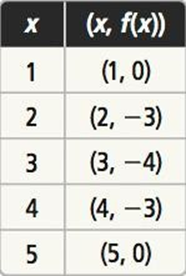Maths-
General
Easy

Question

# Compare each function to f, shown in the table. Which function has lesser minimum value? Explaing(x) = 2x2 + 8x + 3

## The correct answer is: -5

### Solution:- We have given two functions f(x) and g(x).g(x) = 2x2 + 8x + 3For f(x) , minimum value of the function will be the y-coordinate of the given point which has minimum values(1,0) , (2,-3) , (3,-4) , (4,-3) (5,0)In the given points minimum value of is (3,-4)So, the ,minimum value of f(x) is -4.In g(x)= 2x2 + 8x + 3,  a= 2, b= 8, and c=3. So, the equation for the axis of symmetry is given byx = −(8)/2(2)x = -8/4x = -2The equation of the axis of symmetry for g(x)= 2x2 + 8x + 3 is x = -2.The x coordinate of the vertex is the same:h =-2The y coordinate of the vertex is :k = f(h)k = 2h2 + 8h + 3k = 2(-2)2 + 8(-2) + 3k = 8 - 16 + 3k = -5Therefore, the vertex is (-2 , -5)The minimum value of g(x) will be the y-coordinate of vertex = -5#### With Turito Foundation.#### Get an Expert Advice From Turito.